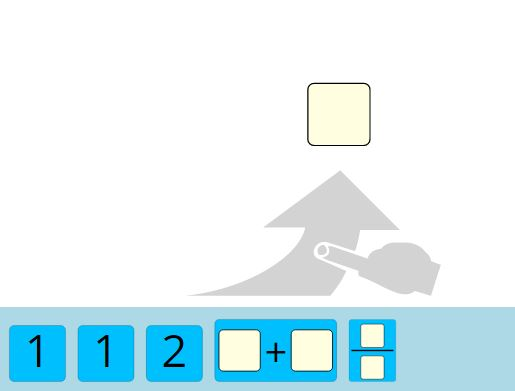There are three steps in creating an exercise.

2. Creating the solution
3. Choosing an interactive solution method

To add a new exercise you have to click on the down arrow on an exercise topic and choose "Add content". Then you can decide between:

• text-exercise
• text-exercise-group
• math puzzle

There is an guidelinie on how to express a valuable task.

### Text-exercise

If you want to have an exercise with a single task you choose "text-exercise".

If you have chosen the text-exercise you can insert the exercise task in "content". With the editor you have multiple methods to arrange and design your task.

Example: text-exercise

### Text-exercise-group

If you want to have subtasks you have to choose "text-exercise-group"

Example: text-exercise-group

### Math puzzle

Example: Math puzzle

insert Example here

If you want to create a Math puzzle you have to choose "Math puzzle" and create the task with the editor. There are no further steps neccesary to create a solution.

Now insert the exercise task in "content".

You can set the end result and the movable elements in "Source code" field. You can do this with the following syntax:

• First type in end result
• Then type in an equality sign "="
• Last you can insert the movable elements sepratet with spaces
(A list of available elements is found below)

#### Example:

With the source code: "1.5 = 1 1 2 + /" you will get the elements on the right.

To solve this puzzle the user has to build a term with the end result $1.5$.#### List of available elements:

• Numbers (e.g.: $1;\;2;\;3;\;-2;\;2.3;\;12.7$)
• Operators: $+\;-\;*\;/ \;$ ^
• The constant $\pi$: $pi$
• Variables: have to signed with an \$ bevor (e.g.: $x;\;y$)

## 2. Creating the solution

There is an additional guidelinie on how to express the solution of a task.

## 3. Interactive solution methods

Additionally to the text solution you are able to select interactive solutions methods. These let you do a "pre-check" of your answer without reading the whole solution.

There are two different kinds of interactive methods:

a. Single choice method

b. Input challenge

### a. Single choice method

With a single choice method you can add several answer options. One of the options is the right answer and the other ones are wrong.

Example: single choice exercise

To add the right answer you have to click on the down arrow next to the task and select "Add single-choice-right-answer". In "content" you write the correct answer to the task. In "feedback" you can write a personal feedback. If "feedback" is left empty then the standard feedback is "Right.".

### b. Input challenge

With an input challenge method you have the opportunity to do a "quick-check" whether your answer is right. This method creates a box and the solution can be written into this box. There are three kinds of input challenge.

First there is the "Input-string-normalized-match-challenge" which expects any kind of numbers and letters as an input. To add this input challenge you have to select the down arrow next to your ask and choose "Add input-string-normalized-match-challenge". In "content" you write the correct answer and additionally to can add a personal feedback. It is important that the exact solution has to be typed into the box.

Example: input-string-normalized-match-challenge

Second there is "Input-number-exact-match-challenge" which expects a number as an input. It can be added in the same way as the first input challenge.

Example: input-number-exact-match-challenge

Third there is "Input-expression-match-challenge" which expects a mathematical term. This input challenge checks different equivalencies. For example if the right answer is "$2x$", then "$x$*$2$" ist also a right answer. This input challenge can be added in the same way as the first one.

Example: input-expression-match-challenge

If you know some typical mistakes to your task you are able to create a particular input challenge that gives feedback to a wrong answer. To create this you have to click on down arrow next to the existing input challenge. There you have to select a new input-match-challenge. Then you can type the wrong answer and give a personal feedpack to this answer.

Example: Personal feedback for wrong answer

Type "2^5" into the box: